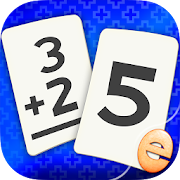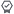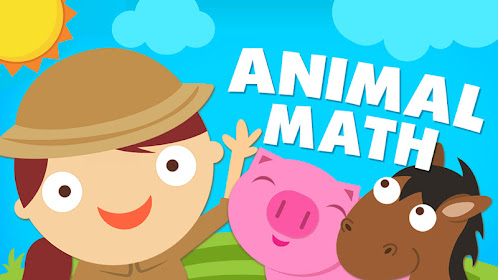Ages 6-8

# Addition Flash Cards Math Help Learning Games FreeEveryone
239
·
Offers in-app purchases
Addition Flash Card Math games include a practice flash card deck and a variety of matching games, speed challenges, and quizzes to encourage math practice with options for preschool, kindergarten, 1st grade, and 2nd grade lessons.

This app adheres to Common Core Standards for math and was designed by parents and teachers. It's a fun to use tool appropriate for preschoolers, first graders, second graders and adults learning to perfect their addition skills.

• Match equations to their answers
• Choose the best number range for your skill level
• "Show Me" option keeps cards face up for easier play

BUILDING MATH SKILLS:
• Hear the numbers and equations as you touch them
• Positive feedback and balloon popping rewards

• Items, numbers and instructions are professionally narrated
• Hints and options allow you to customize difficulty
• Discover new card designs and layouts as you play
• Parental controls for sound, music and links
• We do not collect personal information from our users.

COMMON CORE STANDARDS:

Kindergarten » Operations & Algebraic Thinking

Understand addition as putting together and adding to, and understand subtraction as taking apart and taking from.

• CCSS.Math.Content.K.OA.A.4 For any number from 1 to 9, find the number that makes 10 when added to the given number, e.g., by using objects or drawings, and record the answer with a drawing or equation.

• CCSS.Math.Content.K.OA.A.5 Fluently add and subtract within 5.

Grade 1 » Operations & Algebraic Thinking

Understand and apply properties of operations and the relationship between addition and subtraction.

• CCSS.Math.Content.1.OA.B.3 Apply properties of operations as strategies to add and subtract. Examples: If 8 + 3 = 11 is known, then 3 + 8 = 11 is also known. (Commutative property of addition.) To add 2 + 6 + 4, the second two numbers can be added to make a ten, so 2 + 6 + 4 = 2 + 10 = 12. (Associative property of addition.)

• CCSS.Math.Content.1.OA.C.6 Add and subtract within 20, demonstrating fluency for addition and subtraction within 10. Use strategies such as counting on; making ten (e.g., 8 + 6 = 8 + 2 + 4 = 10 + 4 = 14); decomposing a number leading to a ten (e.g., 13 - 4 = 13 - 3 - 1 = 10 - 1 = 9); using the relationship between addition and subtraction (e.g., knowing that 8 + 4 = 12, one knows 12 - 8 = 4); and creating equivalent but easier or known sums (e.g., adding 6 + 7 by creating the known equivalent 6 + 6 + 1 = 12 + 1 = 13).

Work with addition and subtraction equations.

• CCSS.Math.Content.1.OA.D.7 Understand the meaning of the equal sign, and determine if equations involving addition and subtraction are true or false. For example, which of the following equations are true and which are false? 6 = 6, 7 = 8 - 1, 5 + 2 = 2 + 5, 4 + 1 = 5 + 2.

Use place value understanding and properties of operations to add and subtract.

• CCSS.Math.Content.1.NBT.C.4 Add within 100, including adding a two-digit number and a one-digit number, and adding a two-digit number and a multiple of 10, using concrete models or drawings and strategies based on place value, properties of operations, and/or the relationship between addition and subtraction; relate the strategy to a written method and explain the reasoning used. Understand that in adding two-digit numbers, one adds tens and tens, ones and ones; and sometimes it is necessary to compose a ten.

Grade 2 » Operations & Algebraic Thinking

• CCSS.Math.Content.2.OA.B.2 Fluently add and subtract within 20 using mental strategies. By end of Grade 2, know from memory all sums of two one-digit numbers.

Use place value understanding and properties of operations to add and subtract.

• CCSS.Math.Content.2.NBT.B.5 Fluently add and subtract within 100 using strategies based on place value, properties of operations, and/or the relationship between addition and subtraction.
Collapse

Review Policy
3.8
239 total
5
4
3
2
1

## What's New

Performance improvements. If you love our app, please rate or review it. Thank you!
Collapse

Updated
August 6, 2020
Size
47M
Installs
100,000+
Current Version
2.5.0
Requires Android
4.0.3 and up
Content Rating
Everyone
Interactive Elements
Digital Purchases
In-app Products
\$3.99 per item
Permissions
Offered By
Eggroll Games
Developer
Eggroll Games LLC 826 Bitting Hall Dr Rural Hall, NC 27045